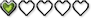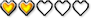# Controllable Conveyors

2 replies to this topic

### #1Korben

Korben

Junior

•• Members
•• Gender:Unspecified
• Location:USA

Posted 29 July 2022 - 02:42 PM

Would any one be able to make a script that changes the direction of conveyor combos when Link changes direction? So if you press right the conveyors will become right-facing conveyors and if you press left they will become left-facing etc. This would preferably be usable with conveyors combos on the same screen that are static and don't change direction, so you could have both controllable conveyors and static conveyors on one screen.

### #2Emily

Emily

Scripter / Dev

•• ZC Developers
•• Gender:Female

Posted 29 July 2022 - 08:25 PM

```combodata script controllableConveyor
{
//Set up the conveyor speed as normal, the script will make it change directions
//Set the combo to an up-facing conveyor graphic, with 0 flip
//The graphic will be flipped vertically for down-facing,
// rotated 90° for right-facing,
// and flipped horizontally from the right-facing for left-facing
void run()
{
int speed = 2;
int rate = 3;
if(this->Flags)
{
if(this->Attributes) //Horizontal?
{
if(this->Attributes) //diagonal?
{
speed = Sqrt(Pow(this->Attributes,2) + Pow(this->Attributes,2));
}
else speed = Abs(this->Attributes);
}
else if(this->Attributes) //Vertical?
speed = Abs(this->Attributes);
}
this->Flags = true;
this->Attribytes = rate;
this->Attributes = 0;
this->Attributes = 0;

while(true)
{
switch(Hero->Dir)
{
case DIR_UP:
this->Attributes = 0;
this->Attributes = -speed;
this->Flip = 0;
break;
case DIR_DOWN:
this->Attributes = 0;
this->Attributes = speed;
this->Flip = 2;
break;
case DIR_LEFT:
this->Attributes = -speed;
this->Attributes = 0;
this->Flip = 5;
break;
case DIR_RIGHT:
this->Attributes = speed;
this->Attributes = 0;
this->Flip = 4;
break;
}
int dir = Hero->Dir;
while(dir == Hero->Dir)
Waitframe();
}
}
}

```

Relatively simple script, even handles rotating/flipping the tiles for you. Requires 2.55. Just assign the script to an up-facing conveyor, and if you want to set the speed, use the "Custom Speed" flag and enter the custom speed as normal for non-scripted conveyors.

Other conveyors without the script assigned will not be affected.

• Korben likes this

### #3Korben

Korben

Junior

•• Members
•• Gender:Unspecified
• Location:USA

Posted 30 July 2022 - 04:33 PM

```combodata script controllableConveyor
{
//Set up the conveyor speed as normal, the script will make it change directions
//Set the combo to an up-facing conveyor graphic, with 0 flip
//The graphic will be flipped vertically for down-facing,
// rotated 90° for right-facing,
// and flipped horizontally from the right-facing for left-facing
void run()
{
int speed = 2;
int rate = 3;
if(this->Flags)
{
if(this->Attributes) //Horizontal?
{
if(this->Attributes) //diagonal?
{
speed = Sqrt(Pow(this->Attributes,2) + Pow(this->Attributes,2));
}
else speed = Abs(this->Attributes);
}
else if(this->Attributes) //Vertical?
speed = Abs(this->Attributes);
}
this->Flags = true;
this->Attribytes = rate;
this->Attributes = 0;
this->Attributes = 0;

while(true)
{
switch(Hero->Dir)
{
case DIR_UP:
this->Attributes = 0;
this->Attributes = -speed;
this->Flip = 0;
break;
case DIR_DOWN:
this->Attributes = 0;
this->Attributes = speed;
this->Flip = 2;
break;
case DIR_LEFT:
this->Attributes = -speed;
this->Attributes = 0;
this->Flip = 5;
break;
case DIR_RIGHT:
this->Attributes = speed;
this->Attributes = 0;
this->Flip = 4;
break;
}
int dir = Hero->Dir;
while(dir == Hero->Dir)
Waitframe();
}
}
}

```

Relatively simple script, even handles rotating/flipping the tiles for you. Requires 2.55. Just assign the script to an up-facing conveyor, and if you want to set the speed, use the "Custom Speed" flag and enter the custom speed as normal for non-scripted conveyors.

Other conveyors without the script assigned will not be affected.

This is great, thank you.

#### 1 user(s) are reading this topic

0 members, 1 guests, 0 anonymous users